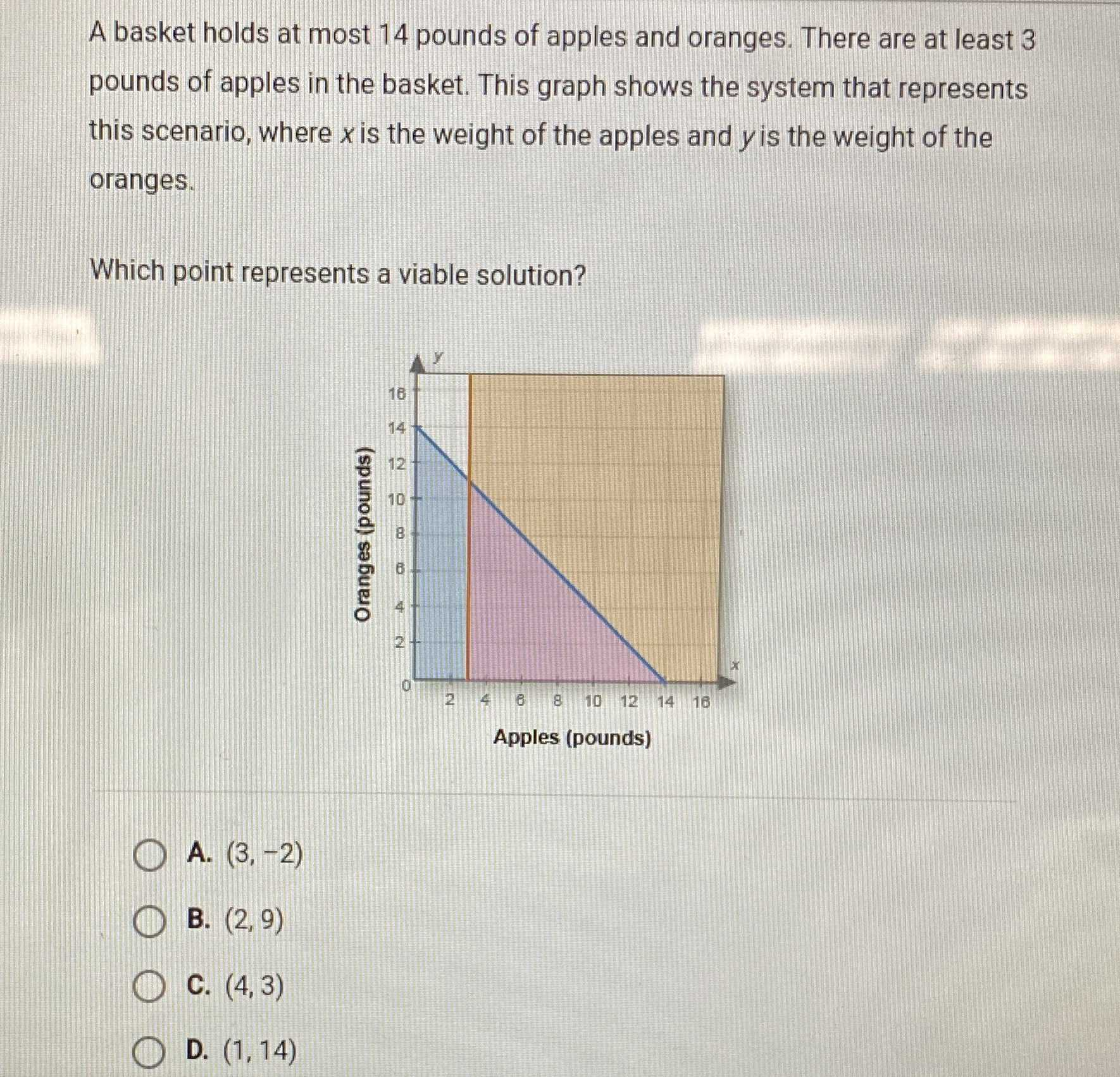### ¿Todavía tienes preguntas de matemáticas?

Pregunte a nuestros tutores expertos
Algebra
PreguntaA basket holds at most $$14$$ pounds of apples and oranges. There are at least $$3$$ pounds of apples in the basket. This graph shows the system that represents this scenario, where $$x$$ is the weight of the apples and $$y$$ is the weight of the oranges. Which point represents a viable solution? A. $$( 3 , - 2 )$$

B. $$( 2,9 )$$ C. $$( 4,3 )$$ D. $$( 1,14 )$$